#### Howdy, Stranger!

It looks like you're new here. If you want to get involved, click one of these buttons!

# Another competition, a long time ago, in a galaxy far away...

edited December 2015 Posts: 5,396

What else could it be but Star Wars?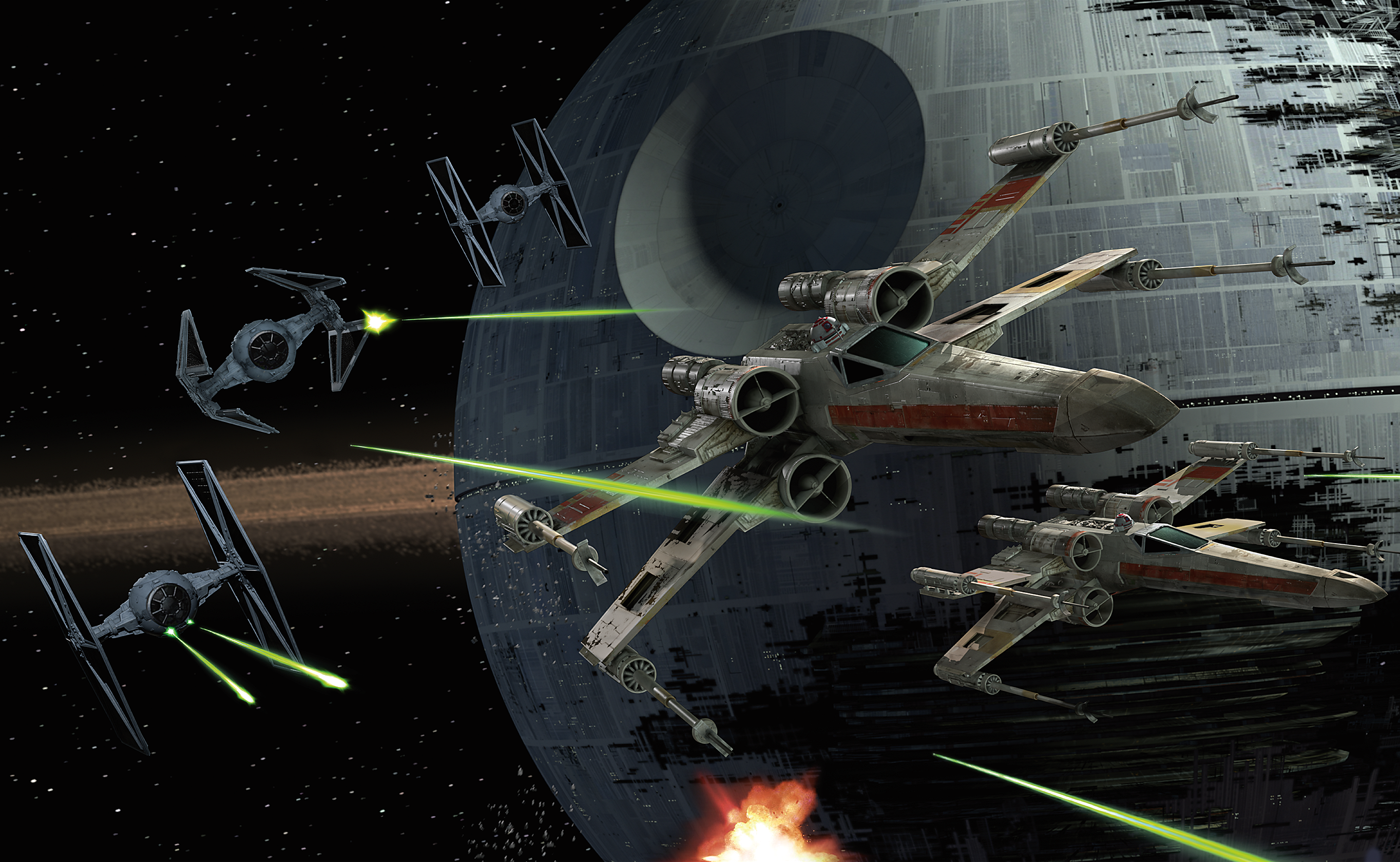It doesn't have to be fancy or complicated, just make it fun. So whether you're a beginner or an expert, have a go.

No limits.

Deadline is the end of the year.

«13

• Posts: 5,396

I'm entering this one myself, so someone else can be the judge!

• edited December 2015 Posts: 891

We have to programm a game? Forget me! Leave me a judge, it's better then developing!

• Posts: 5,396

It doesn't have to be a game. It can be anything you like, as long as it has something to do with Star Wars.

• Posts: 891

Has the code be short?

• Posts: 5,396

No, any length

• Posts: 891

I don't know how to make pictures online, can I send the picture you to your mail, and you will send it here?

• Posts: 9,298

@TokOut To get/use a picture, use the sprite() command. Tap inside the () and from the Documents or Dropbox folder, use the `add from photos` option.

• Posts: 891

@dave1707 , I mean if the project is ready pictures to Photos

• edited December 2015 Posts: 891

https://gist.github.com/TokOut/94445bfe193362c22a28 - here is an example but it doesnt count

• edited December 2015 Posts: 884

Laying down some concepts. Boba fett sent to tatooine/Jakku to retrieve R2D2 & C3PO. Jawas and sand people look on.

• Posts: 5,396

wowee, cool

• Posts: 2,020

@West love the parallax scrolling!

• Posts: 18

@west those are some great effects! The scrolling is great

• edited December 2015 Posts: 226

Edit: My contribution. Best wishes to all of you(I used the laser beam of Jedi @dave1707)

• edited December 2015 Posts: 5,396

For anyone intimidated by West's great effort, I remind you this competition is not really about winning, it is about having fun with Star Wars, and you can post as many as you like, as simple as you like.

Here is my warm up, the scrolling text.

I simply drew the text onto a picture which I put on a simple rectangle mesh, used the perspective command to draw in 3D, and made the text move forward at an angle.

• Posts: 226

@Ignatz, lol. Should the contribution be just a video? Or the code? Or both?

• Posts: 5,396

whatever you like - although I think a video is often best because people can easily see what you've done. If you think other people will enjoy your code, post the code, or a link.

• Posts: 226

All right, thanks, I'll better post the video.

• edited December 2015 Posts: 9,298

Here's my version.

Code removed. See the updated version below.

• Posts: 5,396

Very nice!

And the next scene follows (I did this some time ago, it has sound too), it's called Luke's nightmare...

• Posts: 5,651

Here's my contribution, a lightsaber effect.

The basic algorithm extrudes a line of points (i.e., the blade) through previous transforms. You can use two fingers to swing the lightsaber around by its handle.

I tried to enhance the appearance through shaders, textures and blend modes.

• Posts: 2,020

I haven't seen Force Awakens yet, but if that's the actual title crawl @dave1707 should add a SPOLIER ALERT to his post!

• Posts: 5,396

I'll have my light saber in blue, thank you. Great effect!

You can make some very simple games using TIE fighters because they are just a circle with a vertical line on each side for the wing. Below is some very basic code for making a bunch of TIEs fly toward the camera, see the video. Feel free to use any of it, if it helps you make something, eg a game where you have to touch them to destroy them before they reach you. You could also use these in a side scrolling or top scrolling arcade shooter game.

``````function setup()
--make a basic TIE pic, 300 pixels square
size=300
tie=MakeTiePic(size)
distance=60 --starting distance
speed=0.1 --per frame
ties={} --create 50 TIEs at random positions
for i=1,50 do
ties[i]=vec3(math.random(WIDTH),math.random(HEIGHT),distance+math.random(100))
end
end

function draw()
background(0, 0, 0, 255)
for i=#ties,1,-1 do --loop through backwards because we may remove some of the,
if ties[i].z<=0 then table.remove(ties,i) --remove them if they get past us
else sprite(tie,ties[i].x,ties[i].y,size/ties[i].z) --otherwise draw them
--(we divide size by distance to adjust for perspective)
end
end
end

function MakeTiePic(s) --makes a TIE image you can use in a game
local img=image(s,s)
setContext(img)
pushStyle()
stroke(150,150,150)
strokeWidth(s/25)
line(0,0,0,s)
line(s,0,s,s)
line(0,s/2,s,s/2)
ellipse(s/2,s/2,s/2)
fill(0)
ellipse(s/2,s/2,s/4)
popStyle()
setContext()
return img
end
``````
• edited December 2015 Posts: 9,298

@yojimbo2000 I haven't seen the movie yet, so I don't know if that's correct or not. I did a Google search for Episode VII and found those words. I guess someone who's seen the movie can say.

EDIT: Actually, I think the last and only Star Wars movie I saw was the original one. VII is playing at the local theater, I might go see it Monday, or not.

• edited December 2015 Posts: 9,298

When I made this yesterday, I didn't have time to include the stars in the background before I went to bed. Here's my updated version.

EDIT: Removed code. A see an updated version below after I saw the movie.

• Posts: 1,255

Ignatz,

I was thinking of reproducing the old Atari 2600 Star Raiders, which was after all based after Star Wars space combat.

• Posts: 5,396

@Mark - do it!

• Posts: 891
• Posts: 5,396

So we're sneaking through this asteroid belt to avoid the Imperials....

• Posts: 103

does it need to be a game, or can it be anything starwars/ space related?

• edited December 2015 Posts: 5,396

there are just two rules -

1. make something to do with Star Wars, and

2. have fun doing it

• Posts: 9,298

Just saw the movie. \$4 tickets and the theater was about 1/10 full. Last Star Wars I saw was the first one in 1977. The original actors sure looked their age in this one. Of course so do I, so I guess it evens out.

• Posts: 2,020

\$4?? Is that USD?

• Posts: 5,396

I think you must have gone to Phantom Menace by mistake (and you were overcharged).

• Posts: 9,298

@yojimbo2000 Yes, \$4 USD. Normally when we go to the show, there's only about 4 or 6 other people, so it seemed crowded at 1/10 full.

• edited December 2015 Posts: 9,298

Here's the opening crawl as well as I remember after seeing the movie. I still might not have the correct amount of words per line, but the color might be close.

``````displayMode(FULLSCREEN)
supportedOrientations(LANDSCAPE_ANY)

function setup()
dist=-1060
stars={}
for z=1,500 do
table.insert(stars,vec3(math.random(WIDTH),
math.random(HEIGHT),math.random(2,3)))
end
end

function draw()
background(0, 0, 0, 255)
translate(0,0,-10)
fill(255)
for a,b in pairs(stars) do
ellipse(b.x,b.y,b.z)
end
perspective(90)
camera(0,-500,200,0,0,0,0,1,0)
dist=dist+.3
fill(255, 199, 0, 255)
text("STAR WARS",0,dist+600)

text("Episode VII",0,dist+500)
text("THE FORCE AWAKENS",0,dist+470)

text("Luke Skywalker has vanished.",0,dist+370)
text("In his absence, the sinister",0,dist+340)
text("FIRST ORDER has risen",0,dist+310)
text("from the ashes of the Empire",0,dist+280)
text("and will not rest until Skywalker, the last Jedi,",0,dist+250)
text("has been destroyed.",0,dist+220)

text("With the support of the REPUBLIC,",0,dist+120)
text("General Leia Organa leads a brave RESISTANCE.",0,dist+90)
text("She is desperate to find her",0,dist+60)
text("brother Luke and gain his",0,dist+30)
text("help in restoring peace and",0,dist)
text("justice to the galaxy.",0,dist-30)

text("Leia has sent her most daring",0,dist-130)
text("pilot on a secret mission",0,dist-160)
text("to Jakku, where an old ally",0,dist-190)
text("has discovered a clue",0,dist-220)
end
``````
• Posts: 884

@Ignatz @kylewood & @yojimbo2000 - thx!

Here's the code plus a couple of images for an updated version. You can now blast the targets on the ground (tap below ground level to shoot). Double tap to activate the tractor beam. No game engine (yet).

Here's a preview video

Rename this to spritesheet and add it to the project
http://imgur.com/Oq17gyY

Rename this to backdrop and add it to the project
http://imgur.com/6PsnJCK

``````-- Codea competition 2015
-- by West
displayMode(FULLSCREEN)
-- Use this function to perform your initial setup
function setup()
createImages()
ground=100
building={}
for i=1,40 do
table.insert(building,{x=math.random(WIDTH),w=20+10*math.random(3),h=60+10*math.random(9),type=math.random(2),t=math.random(2)})
end
house={} --foreground buildings
for i=1,10 do
table.insert(house,{x=math.random(3*WIDTH),y=ground+28,type=math.random(3)})
end

spd=0
torigspd=0
hspd=0
hanim=1
angle=-10
hx=200
hy=HEIGHT/2
tactive=0
torig=vec2(0,0)
wave=1
waveframe={1,2,1,3}
man={}
table.insert(man,{x=math.random(5000),y=ground+12,wave=math.random(4),lift=0,type=1,a=0,vx=0,vy=0,rotspd=0,active=1})
table.insert(man,{x=math.random(5000),y=ground+12,wave=math.random(4),lift=0,type=2,a=0,vx=0,vy=0,rotspd=0,active=1})
for i=1,20 do
table.insert(man,{x=math.random(5000),y=ground+12,wave=math.random(4),lift=0,type=2+math.random(3),a=0,vx=0,vy=0,rotspd=0,active=1})
end
--   person={"c3po","r2d2","sand","jawa","storm"}
laser={}
smoke={}
gunangle=0
gunxoff=7
gunyoff=-36
damage=0
end

function draw()

--damage
if math.random(200)<damage then
end

if spd>10 then spd=10 end
if spd<-3 then spd=-3 end
angle=-spd*3.5
--colors

sprite("Project:backdrop",WIDTH/2,HEIGHT/2,WIDTH,HEIGHT)
--damage bar
fill(0,100)
rect(0.1*WIDTH,0.94*HEIGHT,0.8*WIDTH,0.02*HEIGHT)
local barlen=((100-damage)/100)*WIDTH*0.78
fill(238, 230, 5, 255)
rect(0.101*WIDTH,0.941*HEIGHT,barlen,0.018*HEIGHT)

noStroke()

for i,b in pairs(building) do
if b.type==1 then
sprite(mountainimg[b.t],b.x,130,64,64)
b.x = b.x - spd*b.type/4
if b.x<-100 then b.x=WIDTH+100 end
if b.x>WIDTH+100 then b.x=-100 end
end
end

for i,b in pairs(building) do
if b.type==2 then
sprite(mountainimg[b.t],b.x,130,64,64)
b.x = b.x - spd*b.type/4
if b.x<-100 then b.x=WIDTH+100 end
if b.x>WIDTH+100 then b.x=-100 end
end
end
fill(186, 99, 26, 255)
rect(0,0,WIDTH,ground)
fill(216, 162, 17, 255)
rect(0,ground-9,WIDTH,10)

pushMatrix()
rotate(angle)
sprite(beam,0,0,12,12)
else
sprite(beam,0,0,8,8)
end
popMatrix()
end
--draw slave ship

pushMatrix()
translate(hx,hy)
rotate(angle)
sprite(slave,0,0,156/4,360/4)
popMatrix()

--gun
pushMatrix()
translate(hx,hy)

rotate(angle)
translate(gunxoff,gunyoff)
rotate(math.deg(gunangle))
sprite(gun,0,0,64/4,64/4)
popMatrix()
hanim = hanim + 0.5
if hanim>3.99 then hanim=1 end

hy = hy + hspd
if tactive==0 then
if math.abs(hspd)>0 then
hspd=(math.abs(hspd)-0.03)*hspd/math.abs(hspd)
end

if math.abs(spd)>0 then
spd=(math.abs(spd)-0.02)*spd/math.abs(spd)
end

end
end
for i,m in pairs(man) do
if m.lift==0 then
m.lift=1
end
end
end
end

end

if hy>HEIGHT-20 then hy=HEIGHT-20 end
if hy<ground+20 then hy=ground+20 end
end
for i,h in pairs(house) do
sprite(houseimg[h.type],h.x,h.y)
h.x = h.x - spd
end
for i,m in pairs(man) do

if m.x>0 and m.x<WIDTH and math.random(200)==1 and m.type>2 then
local ang=math.atan(hy-m.y,hx-m.x)
table.insert(laser,{x=m.x,y=m.y,active=1,a=ang})
end

if m.lift==0 then
pushMatrix()
translate(m.x,m.y)
rotate(m.a)
if m.active==2 then
sprite(person[m.type+5],0,0,32,32)
else
sprite(person[m.type],0,0,32,32)
end
popMatrix()
m.x = m.x - spd
m.a = m.a + m.rotspd
m.x = m.x + m.vx
m.y = m.y + m.vy
--only do this if flying
if m.active==2 then
m.vy = m.vy - 0.1
end
if m.y<0 then m.active=0 end
elseif m.lift==1 then
else
m.lift=2 --inside heli
end
end
end

for i,b in pairs(laser) do
pushMatrix()
translate(b.x,b.y)
rotate(-90)
rotate(math.deg(b.a))
sprite("Space Art:Green Bullet",0,0,6,18)
popMatrix()
if math.abs(hx-b.x)<20 and math.abs(hy-b.y)<20 then
damage = damage + 1
b.active=0
end

b.x = b.x + 10*math.cos(b.a)
b.y = b.y + 10*math.sin(b.a)
b.x = b.x - spd
if b.y>HEIGHT then b.active=0 end
if b.y<ground then
b.active=0

for j,m in pairs(man) do
if math.abs(m.x-b.x)<20 and m.active==1 then
m.rotspd=-5+math.random(110)/10
local vel=5+math.random(5)
m.vx=vel*math.sin(dir)
m.vy=vel*math.cos(dir)
m.active=2
end
end
end
end
for i,b in pairs(laser) do

if b.active==0 then
table.remove(laser,i)
end
end

for i,s in pairs(smoke) do
pushMatrix()
translate(s.x,s.y)
rotate(s.a)
sprite("Cargo Bot:Smoke Particle",0,0,s.rise,s.rise)
popMatrix()
s.x = s.x - spd
s.y = s.y + s.rise/5
s.rise = s.rise + 0.25
s.active=0
end
noTint()
end
for i,b in pairs(smoke) do

if b.active==0 then
table.remove(smoke,i)
end
end
for i,b in pairs(man) do

if b.active==0 then
table.remove(man,i)
end
end
end

function touched(t)
if t.tapCount==2 then
end
end

if tactive==0 then
torig=t
tactive=1
torigspd=spd
end
if t.state==MOVING then
spd=torigspd+(t.x-torig.x)/50
hspd=(t.y-torig.y)/100
end
if t.state==ENDED then
tactive=0
if t.y<ground then

--add in ship rotation - angle
local ga=math.atan(gunyoff,gunxoff)
local gr=math.sqrt(gunyoff*gunyoff+gunxoff*gunxoff)

local gx=hx+gxo
local gy=hy+gyo

local ang=math.atan(t.y-gy,t.x-gx)
table.insert(laser,{x=gx,y=gy,active=1,a=ang})
gunangle=ang
end
end
end

function createImages()
ss=image(384,384)
setContext(ss)
sprite("Project:spritesheet",384/2,384/2,384,384)
setContext()
slave=ss:copy(0,0,192,384)
gun=ss:copy(320,256,64,64)
houseimg={}
houseimg=ss:copy(192,320,64,64)
houseimg=ss:copy(256,320,64,64)
houseimg=ss:copy(320,320,64,64)
mountainimg={}
mountainimg=ss:copy(192,256,64,64)
mountainimg=ss:copy(256,256,64,64)
person={}
person=ss:copy(192,0,32,32)
person=ss:copy(192,32,32,32)
person=ss:copy(192,64,32,32)
person=ss:copy(192,96,32,32)
person=ss:copy(192,128,32,32)
person=ss:copy(224,0,32,32)
person=ss:copy(224,32,32,32)
person=ss:copy(224,64,32,32)
person=ss:copy(224,96,32,32)
person=ss:copy(224,128,32,32)
beam= ss:copy(368,224,16,16)
end
``````
• Posts: 891

I think @West will win!

• Posts: 226

@West = winner.

• Posts: 11

Deadline is the end of the year...

• Posts: 891

That's true! But this idea can't be stolen anymore. If someone will make the graphics better and more 3d and another picture, but this is nearly impossible in 10 days...

• Posts: 884

Here's a very simple physics bb8.

Use the background from above plus here's the head and body sprites

``````-- Bb8
--By West
displayMode(FULLSCREEN)
-- Use this function to perform your initial setup
function setup()
size=100
bbody=physics.body(CIRCLE,size)
bbody.x=WIDTH/2
bbody.y=HEIGHT
physics.body(EDGE,vec2(0,30),vec2(WIDTH,30))
physics.body(EDGE,vec2(0,30),vec2(0,HEIGHT))
physics.body(EDGE,vec2(WIDTH,30),vec2(WIDTH,HEIGHT))
bbody.restitution=0.2
end

-- This function gets called once every frame
function draw()
-- This sets a dark background color
background(40, 40, 50)
sprite("Project:background",WIDTH/2,HEIGHT/2)
noStroke()
fill(163, 82, 30, 255)
rect(0,0,WIDTH,30)
physics.gravity(Gravity)
-- This sets the line thickness
strokeWidth(5)
pushMatrix()
translate(bbody.x,bbody.y)
rotate(bbody.angle)
sprite("Project:bb8body",0,0,2*size)
popMatrix()
end

``````
• Posts: 5,396

setting the standard there, @West!• edited December 2015 Posts: 2,020

Hey everyone, have an X-wing with R2 unit for Christmas!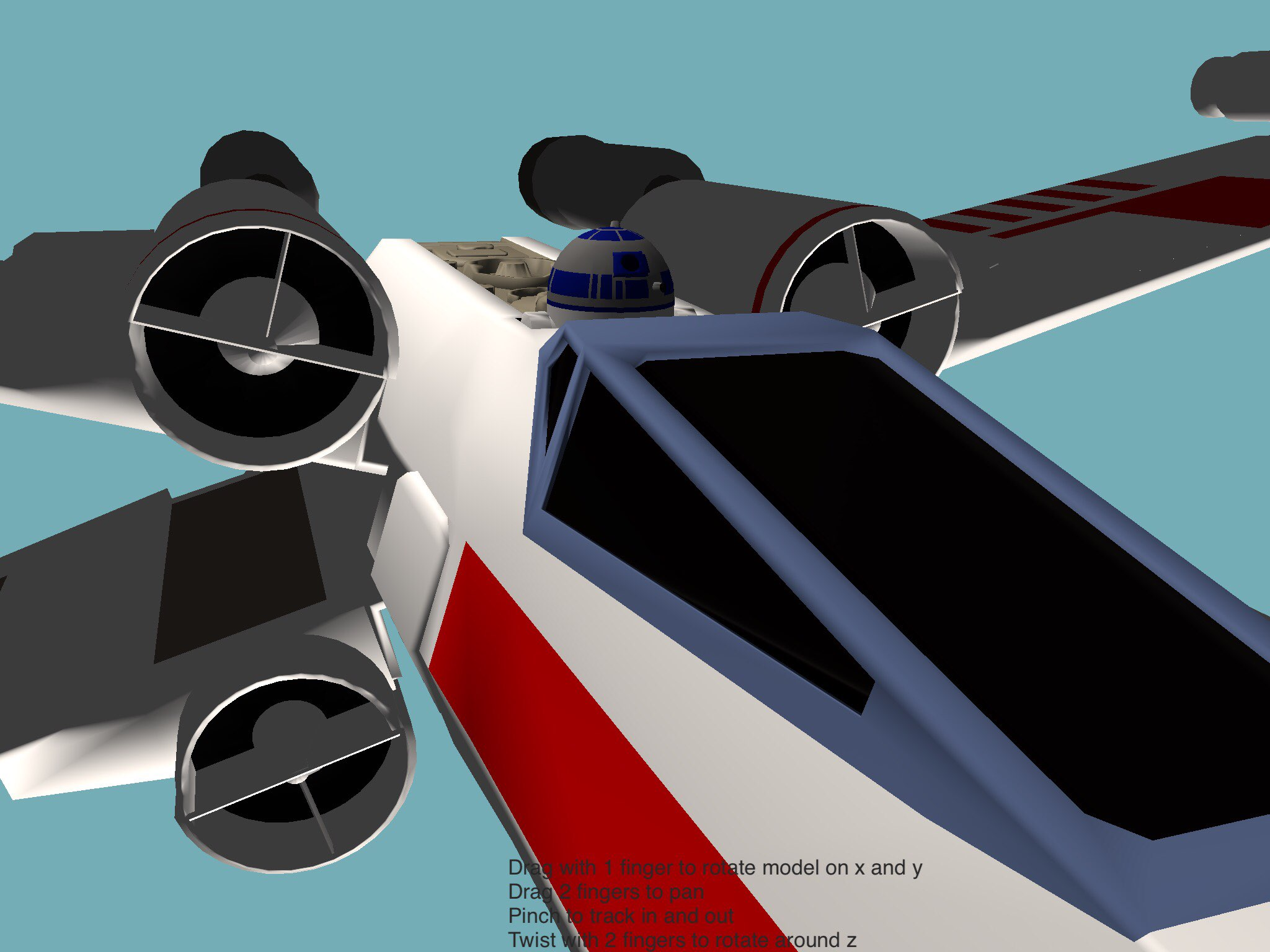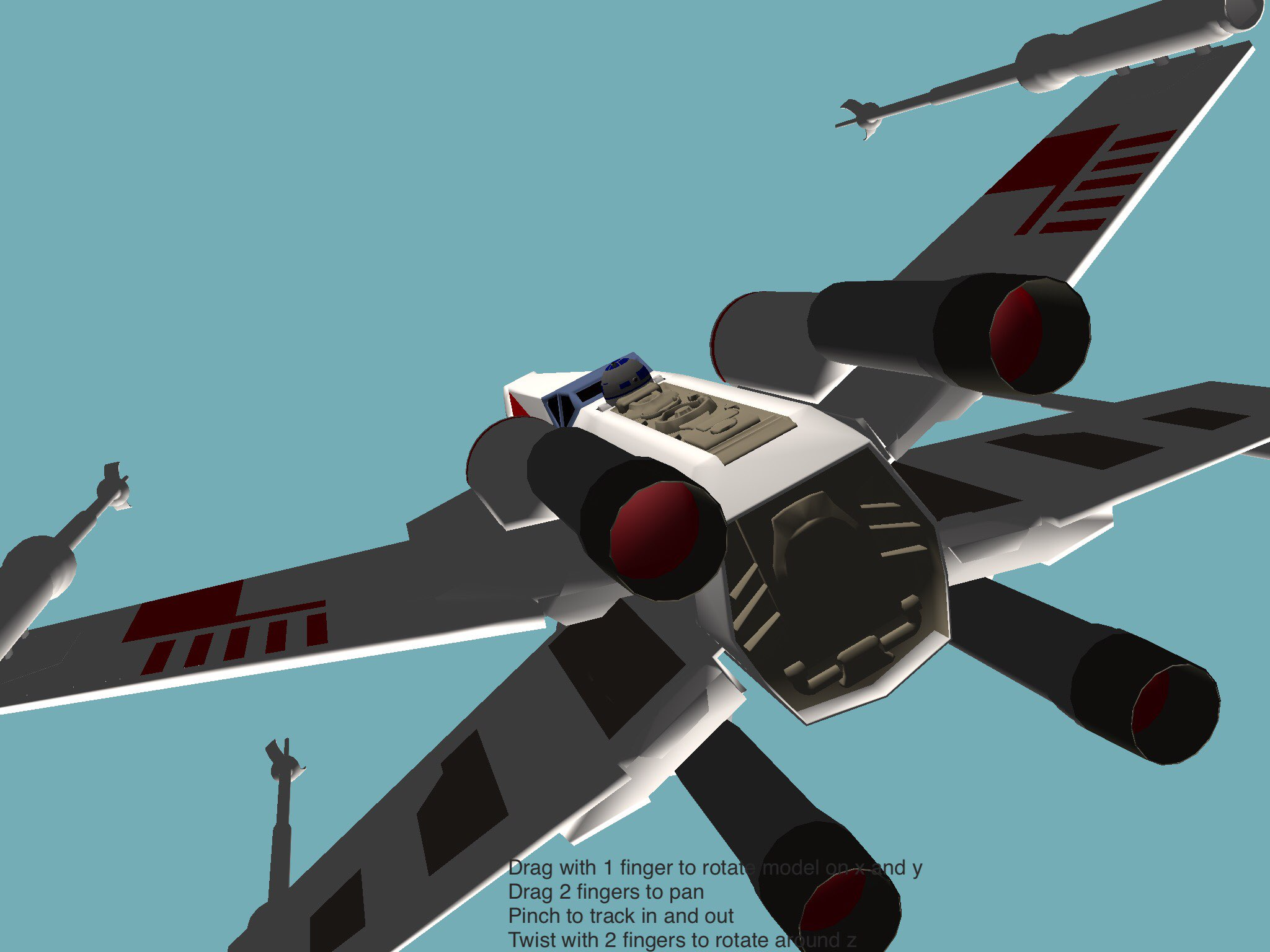Looks pretty sweet in wireframe mode too: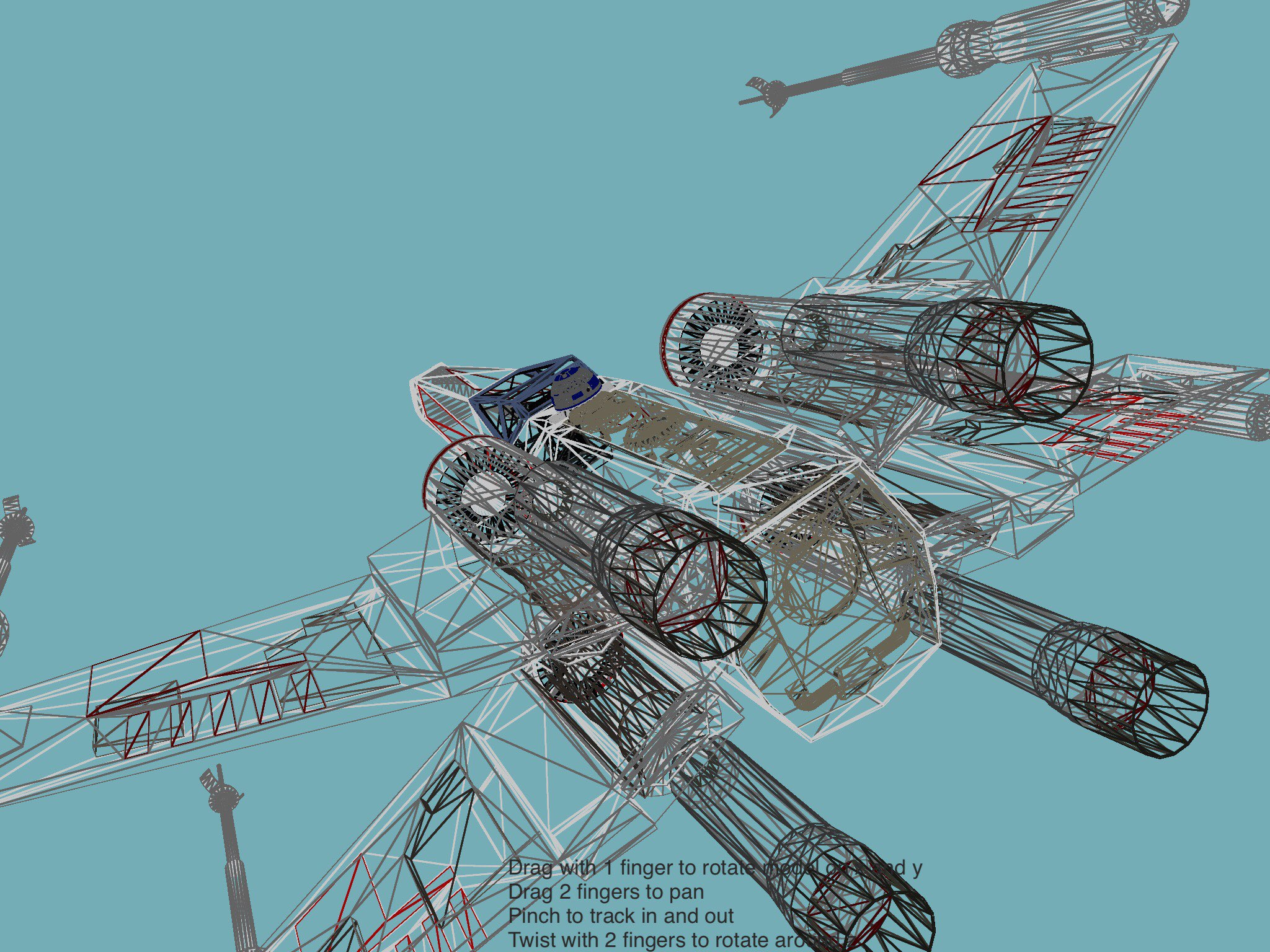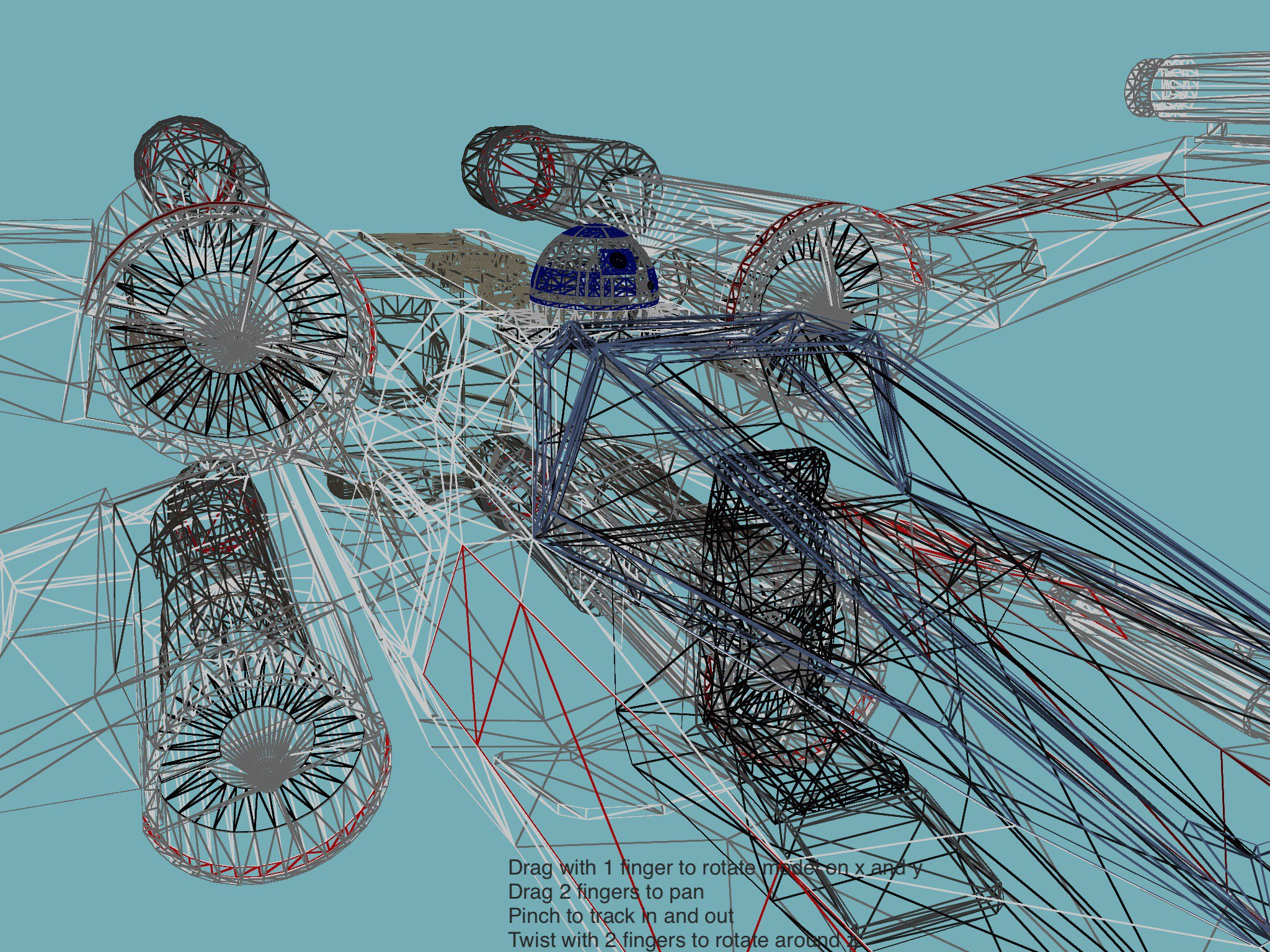Model is a CC-BY licence, so you're free to use it in your projects as long as you credit the creator, PixelOz

http://www.blendswap.com/blends/view/50244

Not sure I'll have time to do much more with this before the end of year deadline. I was thinking of maybe doing an animated storm trooper

• Posts: 5,651

@yojimbo2000 wow, that's pretty awesome. Detailed model. How are you rendering the wire version?

• Posts: 5,396• Posts: 2,020

With a wireframe shader I ported. It uses the standard derivatives GLES 2.0 add-on (which I guess is included as part of the standard library in 3.0):

``````wireframe.vert = [[
uniform mat4 modelViewProjection;
attribute vec4 position;
attribute vec4 color;
attribute vec3 normal;
varying highp vec4 vColor;
varying highp vec3 vNormal;
void main(void) {
vColor = color;
vNormal = normal;
gl_Position = modelViewProjection * position;
}]]

wireframe.frag = [[
#extension GL_OES_standard_derivatives : enable
uniform highp float strokeWidth;
varying highp vec4 vColor;
varying highp vec3 vNormal;
void main(void) {
highp vec4 col = vColor;
if (!gl_FrontFacing) col.rgb *= 0.5; //darken rear-facing struts
highp vec3 d = fwidth(vNormal);
highp vec3 tdist = smoothstep(vec3(0.0), d * strokeWidth, vNormal);
//2 methods: 1. discard method: best way of ensuring back facing struts show through
if (min(min(tdist.x, tdist.y), tdist.z) > 0.5) discard;
else gl_FragColor = mix(col, vec4(col.rgb, 0.), -0.5 + 2. * min(min(tdist.x, tdist.y), tdist.z)); // anti-aliasing

//2. alpha method means some rear faces wont show. Would be good for a "solid" mode though
//gl_FragColor = mix(col, vec4(0.), min(min(tdist.x, tdist.y), tdist.z));
}]]
``````
• Posts: 884
Fab @yojimbo2000 - the force is strong with this one!
• edited December 2015 Posts: 3,297And the winner is...
 just got the code: perfect install, perfect smoothness, perfect rendering...
And the winner is...